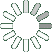Puzzle Command
Daily Puzzle
Number Logic #12
January 18, 2015Find a 6 digit number where:

The 1st digit is 5.
The 2nd digit is a prime number.
The 3rd digit is a prime number.
The 4th digit is the sum of the 1st and 2nd digit.
The 5th digit is the sum of the 1st, 2nd, and 3rd digit.
The 6th digit is 2.

Puzzle Notes
6 digit numbers range from 100,000 to 999,999.
Enter in your solution below and click submit.